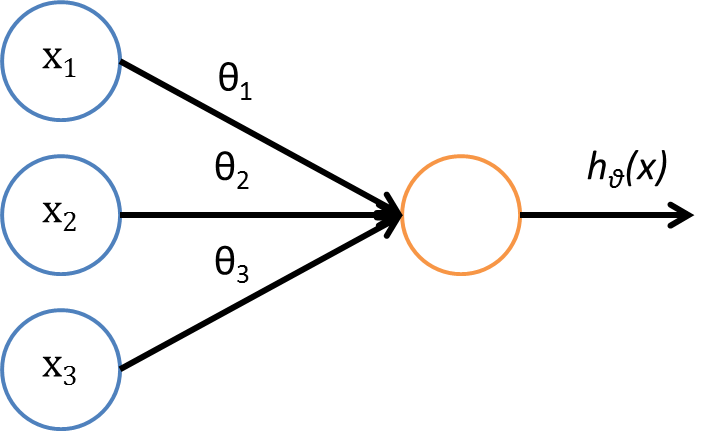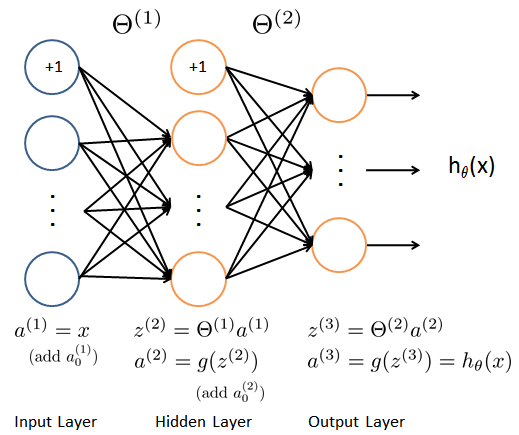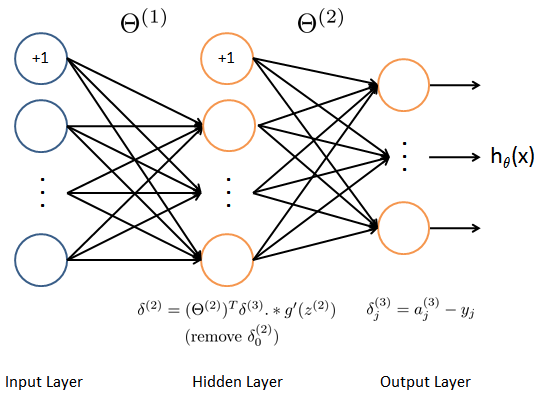# Neural Network.

In coursera, I learned the Neural Network that is a data structure and machine learning algorithm that mimics brain. This is developed from simulation of network of brain neuron. You suppose example of computer vision, you are learning to recognize cars from 100×100 pixel images (not RBG), Let the features be pixel intensity values. If you train logistic regression including all the quadratic terms as features, about how many features will you have ? This answer is 50 million, this means that it's too much features for classification in nonlinear hypothesis. So it doesn't apply to logistic regression. But the Neural Network enable you to clear these complex hypothesis or complex nonlinear hypothesis.

# Neuron Model

The neuron is computation units that takes many inputs and computes from inputs and sends output of computation to other neuron. You can draw below neuron model. In this image, there is notthat is the bias unit, but you can draw this extra note bias unit and sometimes not.This neuron model use the sigmoid activation function as logistic regression.is bias unit that is always 1. θ is weights parameters vector.

# Neural Network and Forward Propagation

In Neural Network, this model is composed from three layer, "Input Layer", "Hidden Layer" and "Output Layer".is "activation" of unit i in layer j.is matrix of weights controlling function mapping from layer j to layer j+1.We can compute this model below:If network hasunits in layer j,units in layer j+1, thenwill be of dimension.

And we can compute neural network model is vectorized implementation. Replace input of logistic function to, as above network image you suppose compute hidden layer. So below is x and z vector:And computebelow:and addIt called "Forward propagation". It start of with the activation of the input-units and then we sort of forward propagation that to the hidden layer and compute the activation of the hidden layer and then we sort of forward propagation that and compute the activation of the output layer, in summary this process of computing the activation from the input then the hidden then output layer.

# Cost Function of Neural Network

First, it define thatis total of number of layers in network andis number of units (not counting bias unit) in layer l. In Neural Network, it make cost function of logistic regression generalize more below:

### Logistic Regression's cost function### Neural Network's cost functionFirst, it calculates sum of number K outputs in this formula. Second is regularization that calculates sum of each elements that squared. You don't need to add i=0 that is bias unit, so i starts from 1. But this is just one possible convention, and even if you were to sum over i equals 0 up tothat would work about the same and doesn't make a big difference.

# Back Propagation Algorithm

As always, we have to make cost function minimum. So we can use "Back Propagation Algorithm" In Neural Network. To compute advanced optimization, it need below code:This calculates partial differential J usingof each of elements of each of layer that sees transition of cost J whenis minor changed. The name of Back Propagation comes from the fact that we start by computing the delta term for the output layer and then we go back a layer and compute the delta terms for the hidden layer and then go back another step to compute any delta and so on. We're sort of back propagating the error from the output layer to other layer.Below is the procedure of back propagation, this run in loop for all training data. First, initializeof each of layer. For important point, you have to assign value which is selected randomly from belowdecided already because it would be fail if it set 0 to all elements in initialization.Second, Run the forward propagation (refer above "Neural Network and Forward Propagation").
Third, Run the back propagation.is "error" of node j in layer l, which is in some sense going to capture our error in activation of that neural network. In back propagation, first step is calculating error on output layer.Next, calculating error on hidden layer below:In this step, ifis sigmoid function,is expressed below:This expression is equal to mathematically the derivative of the g function of the activation function.
Next, add error to matrix delta to accumulates error of each layer of each training sets below:Finally, it divides error delta which is accumulated on each layer by number of training data that means partial differential of θ of j after run all training data.In above formula, it adds normalization to second formula (j≧1) to avoid overfitting.

# Gradient Checking

There is possibility of penetrating bugs into this back propagation because this implement is too complex (especially partial differential of θ of j), so we have to confirm whether this is normal or not using gradient checking. Below formula can be used whenis small enough.If this value closes enough partial differential value of J calculated back propagation, your can confirm that implement is normal. I'm gonna note down this implementation of back propagation:

• Implement backprop to compute DVec (unrolled).
• Implement numerical gradient check to compute gradApprox.
• Make sure they give similar values.
• Turn off gradient checking. Using backprop code for learning.

Important point is that be sure to disable your gradient checking code before training your classifier. If you run numerical gradient computation on every iteration of gradient descent (or in the inner loop of cost function) your code will be very slow.

# Summary, Training a Neural Network

Let's wrap up! I'm gonna summarize above steps to train a neural network.

1. Randomly initialize weights
2. Implement forward propagation to getfor any3. Implement code to compute cost function4. Implement backprop to compute partial derivatives5. Use gradient checking to comparecomputed using backpropagation vs.using numerical estimate of gradient of. Then disable gradient checking code.
6. Use gradient descent or advanced optimization method with backpropagation to try to minimizeas a function of parameters.

That's all.